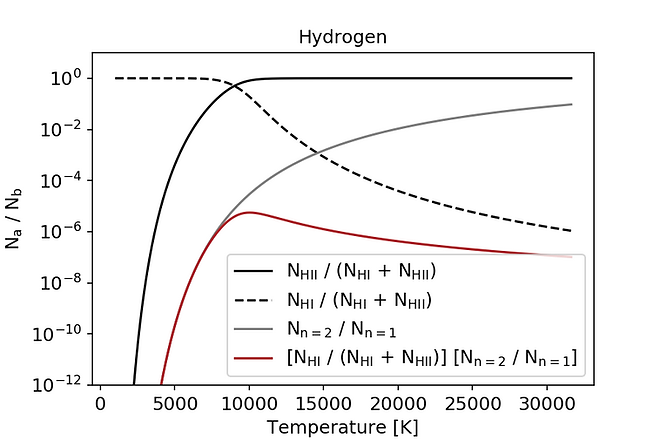# Stellar classification

Stars are historically classified based on absorption lines in the visible part (wavelength between 400 nm and 700 nm) of their spectra. Both the colours of stars and these spectral types (O-B-A-F-G-K-M) are related to the surface temperature of the star. Find below some plots that explain these correlations (colour - temperature - spectral type). For every plot the python script that produces this plot can be downloaded.## Blackbody spectrum

Spectrum for different blackbody temperatures (different colours). Stellar spectral classes (from O to M) are indicated as reference. Our eyes can see light with a wavelength between 400 nm (blue) and 700 nm (red). A star that emits more light at 400 nm than at 700 nm therefore appears blue ("O") and a star that emits more at 700 nm than at 400 nm appears red ("M").

Click here to download the python script to produce this plot (if necessary, change file extension from .txt to .py)## Saha equation and Boltzmann distribution

Stellar spectra are not pure blackbody spectra. At distinct wavelengths the light is absorbed in the outer layers of the star. For hydrogen some absorption lines of the Balmer series have wavelengths that fall into the visible range (400 nm to 700 nm). How strong these absorption lines are depends on how many hydrogen atoms have electrons with a principal quantum number n=2.

The fraction of hydrogen particles that are ionised (H II) and neutral (H I) can be calculated with the Saha equation. Result: Gas with a temperature of > 10,000 K is mostly ionised (black solid line).

The Boltzmann distribution allows one to calculate the relative probability of hydrogen atoms in the different excitation levels. The grey line shows the relative probability of hydrogen atoms with electrons with principal quantum numbers n=2 and n=1. Result: At low temperatures the probability of a hydrogen atom with the excitation level n=2 is very low.

The red line combines the neutral gas fraction (H I, black dashed line) and the probability of an n=2 excitation level. Result: There is a sweet-spot where hydrogen is still mostly neutral but already excited to n=2 around temperatures of 10,000 K. Balmer absorption lines are most prominent for stars with this surface temperature (spectral class "A").

Click here to download the python script to produce this plot (if necessary, change file extension from .txt to .py)